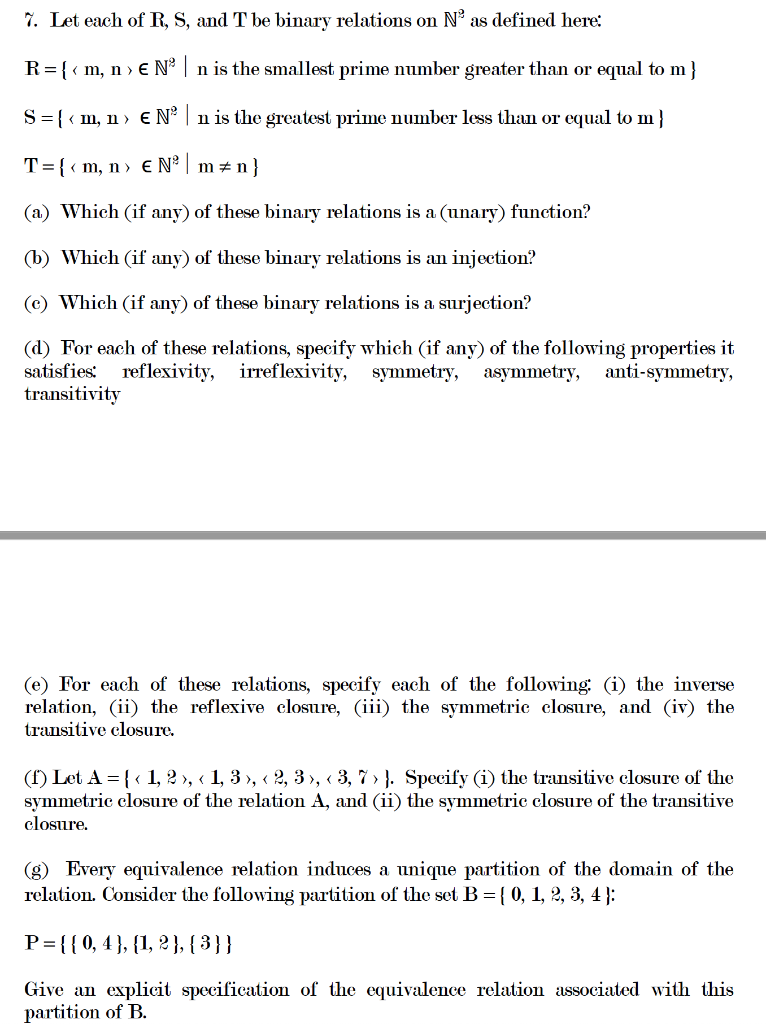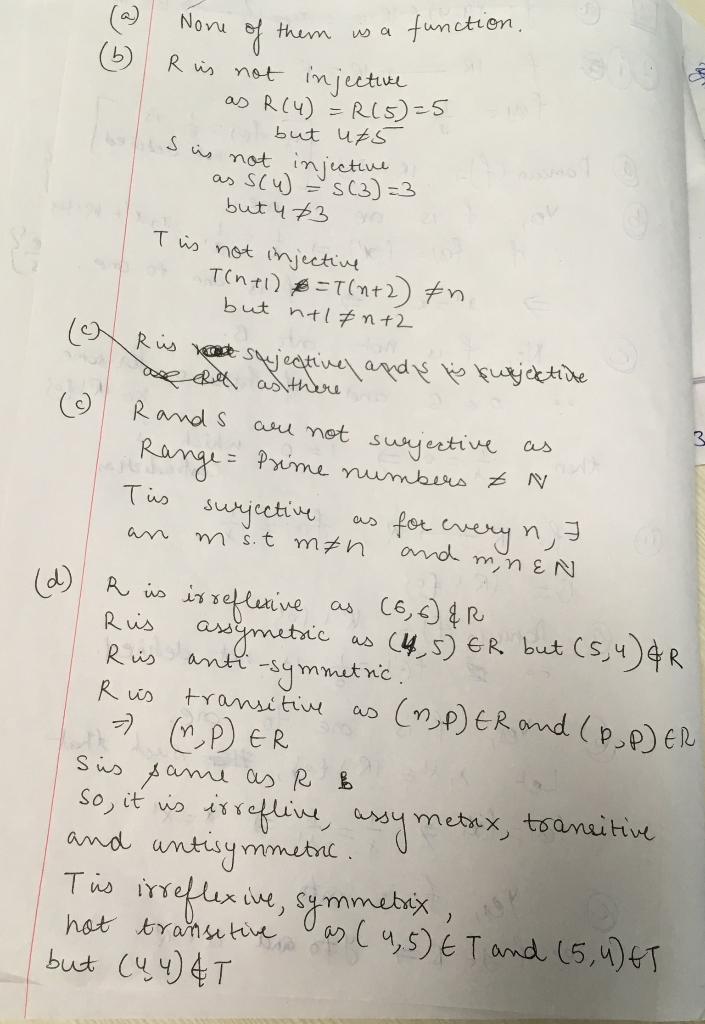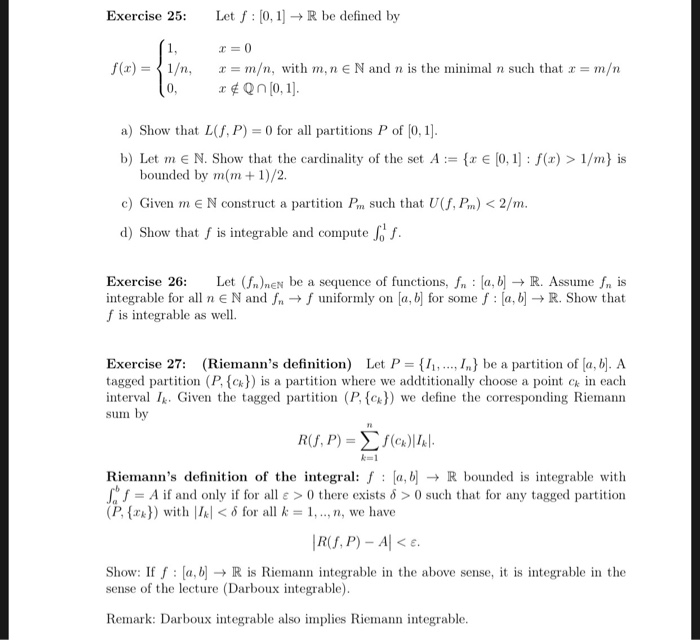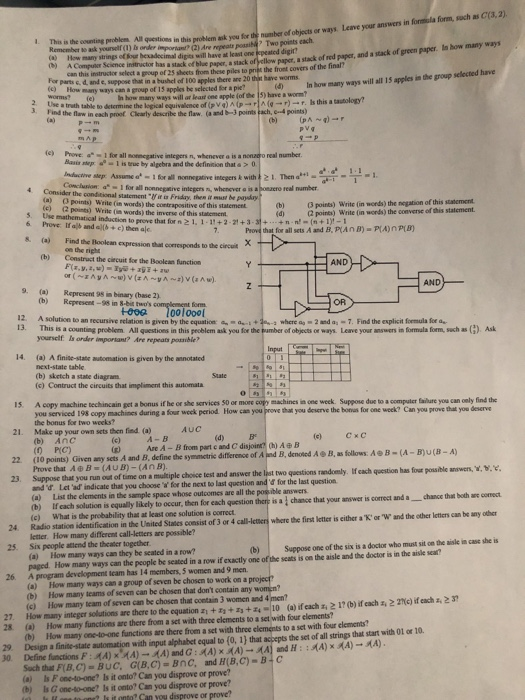# I. Let each of R, S, and T be binary relations on N2 as defined here: R-[<m, n EN nis the smallest prime number greater than or equal to m] S -[< m, n> EN* nis the greatest prime number less...I. Let each of R, S, and T be binary relations on N2 as defined here: R-[we are only supposed to do four parts in a question,please post y

##### Add Answer of: I. Let each of R, S, and T be binary relations on N2 as defined here: R-[<m, n EN nis the smallest prime number greater than or equal to m] S -[< m, n> EN* nis the greatest prime number less...Please all thank you Exercise 25: Let f 0,R be defined by f(x)-1/n, m, with m,nENand n is the minimal n such that m/n a) Show that L(f, P)0 for all partitions P of [0, 1] b) Let mE N. Show that the cardinality of the set A bounded by m(m1)/2. e [0, 1]: f(x) > 1/m) is c) Given m E N construct a partition P such that U(f, Pm)2/m. d) Show that f is integrable and compute Jo...specifically on finite i pmu r the number of objøcts or ways. Leave your answers in fornsiala form, such as C(3, 2) nporkan?(2) Are repeats poasib Two points each imal digits will have at least one xpeated digin? I. This is the oounting problem Al ancmher so ask yourelr (1) ls onder ipo n How many strings of four bexadeci ) A Compuir Science indtructor has a stack of blue can this i For parts c, d. and e, suppose...